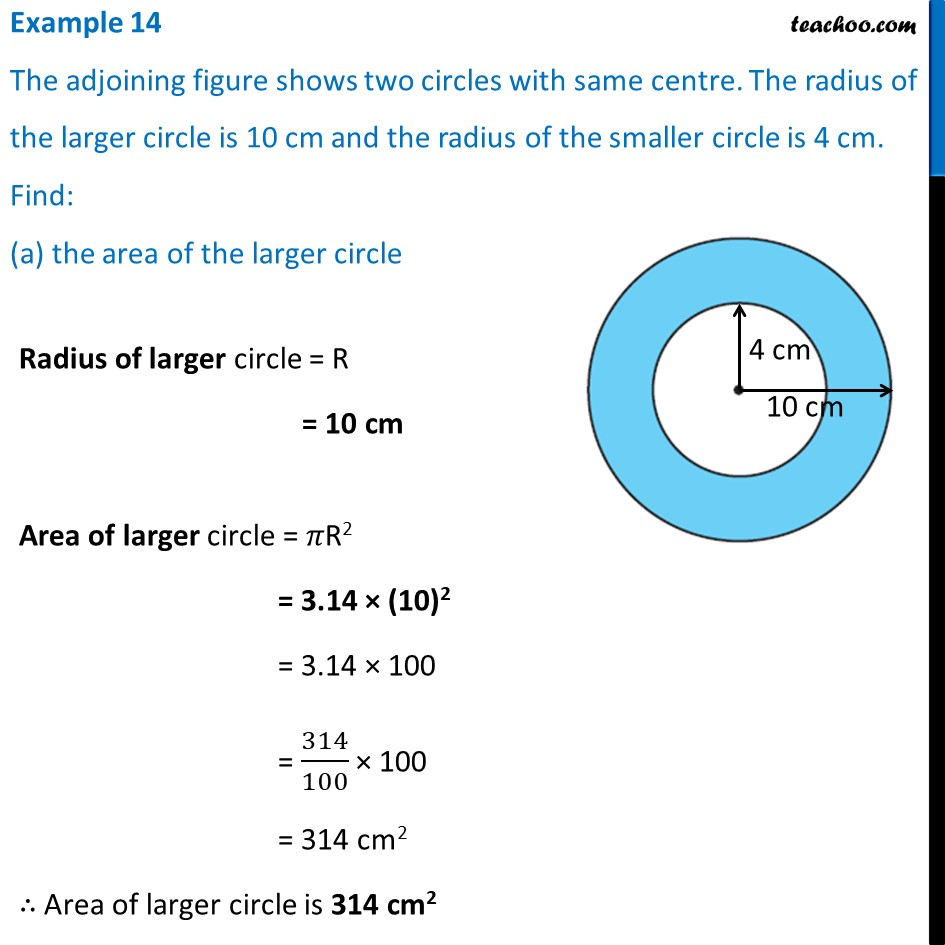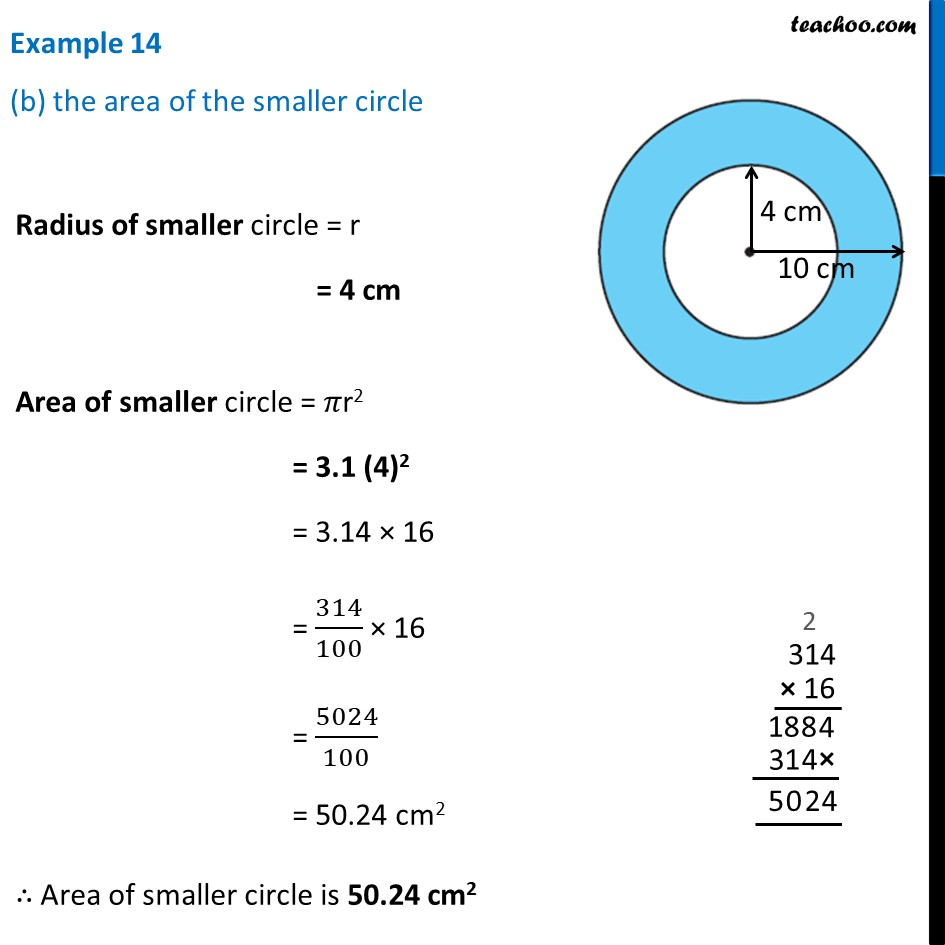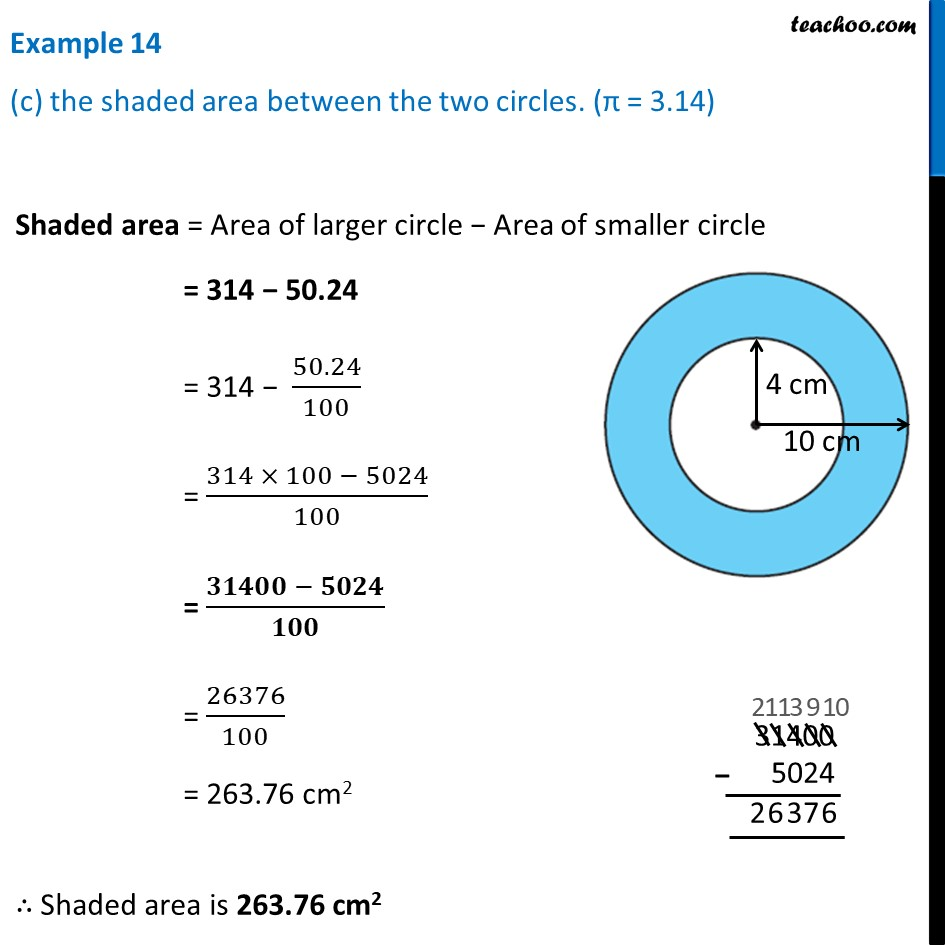Examples

Chapter 9 Class 7 Perimeter and Area
Serial order wiseLearn in your speed, with individual attention - Teachoo Maths 1-on-1 Class

### Transcript

Example 14 The adjoining figure shows two circles with same centre. The radius of the larger circle is 10 cm and the radius of the smaller circle is 4 cm. Find: (a) the area of the larger circle Radius of larger circle = R = 10 cm Area of larger circle = 𝜋R2 = 3.14 × (10)2 = 3.14 × 100 = 314/100 × 100 = 314 cm2 ∴ Area of larger circle is 314 cm2 Example 14 (b) the area of the smaller circle Radius of smaller circle = r = 4 cm Area of smaller circle = 𝜋r2 = 3.1 (4)2 = 3.14 × 16 = 314/100 × 16 = 5024/100 = 50.24 cm2 ∴ Area of smaller circle is 50.24 cm2 Example 14 (c) the shaded area between the two circles. (π = 3.14) Shaded area = Area of larger circle − Area of smaller circle = 314 − 50.24 = 314 − 50.24/100 = (314 × 100 − 5024)/100 = (𝟑𝟏𝟒𝟎𝟎 − 𝟓𝟎𝟐𝟒)/𝟏𝟎𝟎 = 26376/100 = 263.76 cm2 ∴ Shaded area is 263.76 cm2